HLIBpro  2.4
Many Right Hand Sides

For equation systems with many right hand sides, e.g.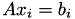with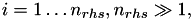different ways are supported in 𝓗𝖫𝖨𝖡𝗉𝗋𝗈 to solve the equations, which shall be introduced in this example.

# Problem Description

We assume a given system matrix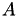and right hand sides (RHSs)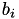. We are looking for the solutions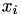in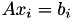.

As with all previous applications of 𝓗-matrices, the 𝓗-LU factorisation shall be used to solve the equation systems. This may either be performed directly, i.e., as a direct solver involving a single matrix-vector evaluation, or with the help of an iterative solver. In all examples so far, the iterative approach was used since it usually relaxes the accuracy demands on the 𝓗-LU factorisation and hence reduces the factorisation runtime.

However, iterative solvers perform several matrix-vector multiplications for a single RHS. Therefore, this approach may increase the overall time significantly for many RHSs.

Alternatively, the direct solver approach may be used, which normally demands a much higher accuracy for 𝓗-LU to obtain the corresponding accuracy in the solution vector. A higher 𝓗-LU accuracy often increases the runtime and memory requirement of the factorisation process. However, depending on the number of RHSs, this additional costs may shorten the overall solution process.

Beside solving for a single RHS, 𝓗𝖫𝖨𝖡𝗉𝗋𝗈 also offers to solve for all RHSs simultaneously when used as a direct solver, as described below.

# Problem Setup

In the following, we use a system matrixand corresponding RHSsfrom the Boundary Element Methods example. However, instead of the Helmholtz kernel, the Laplace kernel is used. Furthermore, the matrix is constructed as an unsymmetric matrix, i.e., with upper and lower triangular part (see below for standard symmetric case).

The initialisation and construction of cluster trees is:

#include <iostream>
#include <string>
#include <boost/format.hpp>
#include <tbb/parallel_for.h>
#include "hlib.hh"
using namespace std;
using namespace HLIB;
using boost::format;
using real_t = HLIB::real;
using fnspace_t = TConstFnSpace;
int
main ( int argc, char ** argv )
{
string gridfile = "grid.tri";
double eps = 1e-4;
double fac_eps = 1e-6;
if ( argc > 1 )
gridfile = argv;
try
{
INIT();
TWallTimer timer;
TAutoBSPPartStrat part_strat;
TBSPCTBuilder ct_builder( & part_strat );
TBCBuilder bct_builder;
auto grid = read_grid( gridfile.c_str() );
auto fnspace = make_unique< fnspace_t >( grid.get() );
auto coord = fnspace->build_coord();
auto ct = ct_builder.build( coord.get() );
auto bct = bct_builder.build( ct.get(), ct.get(), & adm_cond );

This is followed by 𝓗-matrix construction:

slpbf_t bf( fnspace.get(), fnspace.get() );
TBFCoeffFn< slpbf_t > coeff_fn( & bf,
bct->row_ct()->perm_i2e(),
bct->col_ct()->perm_i2e() );
TACAPlus< real_t > lrapx( & coeff_fn );
TTruncAcc acc = fixed_prec( eps );
TDenseMBuilder< real_t > h_builder( & coeff_fn, & lrapx );
auto A = h_builder.build( bct.get(), unsymmetric, acc, & progress );

Here, the unsymmetric matrix is constructed instead of the standard symmetric version.

# RHS Representation

Next, the RHSs shall be computed. For the BEM problem this is accomplished by deriving from TBEMFunction. Since multiple, different RHSs are to be constructed, two parameters alpha and beta are added, which are used in the evaluation function:

class TMyRHS : public TBEMFunction< real_t >
{
private:
const double _alpha, _beta;
public:
TMyRHS ( const double alpha,
const double beta )
: _alpha( alpha )
, _beta( beta )
{}
// actual RHS function
virtual real_t
eval ( const T3Point & pos,
const T3Point & ) const
{
return real_t( sin( 0.5 * pos.x() * cos( _alpha * 0.25 * pos.z() ) ) * cos( _beta * pos.y() ) );
}
};

Up to now, the RHS was represented as a single vector. For multiple RHSs this may be extended to a list of vectors. However, even more compact, and also useful for the different solve methods, is the representation as a dense matrix, i.e., each column corresponds to a different RHS: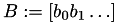In the source code the matrix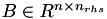is stored in the dense matrix RHS:

const size_t nrhs = 500;
TQuadBEMRHS< fnspace_t, real_t > rhs_build( 4 );
TDenseMatrix RHS( fnspace->n_indices(), nrhs );

For the actual construction, each column of RHS is taken as a standard vector and computed in parallel using tbb::parallel_for :

tbb::parallel_for( size_t(0), nrhs,
[&RHS,&rhs_build,&fnspace] ( const size_t i )
{
TMyRHS rhsfn( 100 * drand48() - 50,
100 * drand48() - 50 );
auto b = rhs_build.build( fnspace.get(), & rhsfn );
auto rhs_i = RHS.blas_rmat().column( i );
BLAS::copy( cptrcast( b.get(), TScalarVector )->blas_rvec(), rhs_i );
} );
RHS.permute( ct->perm_e2i(), nullptr );

The parameters alpha and beta of TMyRHS are chosen randomly. Similar to vectors, also the dense matrix has to be reordered to have the same ordering as the indices in the 𝓗-matrix. The permutation is here only applied to the rows, since they correspond to geometrical indices, while the column index only represents the index of the RHS.

Remarks
For simplicity, we have assumed that the RHS is stored in an object of type TScalarVector, which should be true for almost all cases.

# Solving the Equations

For all methods to solve the above equations, the LU factorisation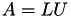is needed, which is computed next:

auto B = A->copy();
TTruncAcc fac_acc( fac_eps, 0.0 );
auto A_inv = factorise_inv( B.get(), fac_acc, & progress );

## Iterative Solver

First, the classical method using an iterative solver is used. For this, each equationis solved in parallel using the solve function. Analogously to the RHSs, the solution vectors are stored in a dense matrix SOL:

{
TDenseMatrix SOL( A->cols(), nrhs );
tbb::parallel_for( size_t(0), nrhs,
[&RHS,&SOL,&A,&A_inv,fac_eps] ( const size_t i )
{
BLAS::Vector< real_t > rhs_i( RHS.blas_rmat().column( i ) );
BLAS::Vector< real_t > sol_i( SOL.blas_rmat().column( i ) );
TScalarVector b( A->row_is(), rhs_i );
TScalarVector x( A->col_is(), sol_i );
solve( A.get(), & x, & b, A_inv.get() );
} );

After all equations are solved, the residual is computed with respect to all RHSs:

auto X = RHS.copy();
multiply( real_t(-1), apply_normal, A.get(), apply_normal, & SOL, real_t(1), X.get(), acc_exact );
cout << " |AX-B| = " << norm_F( X.get() ) << endl;
}

## Direct Vector Solves

Next, the -LU should be used as a direct solver. The only difference to the previous method is, that instead of using the function solve, the function apply of the operator A_inv is used, which applies the inverse ofto the RHS:

{
TDenseMatrix SOL( A->cols(), nrhs );
tbb::parallel_for( size_t(0), nrhs,
[&RHS,&SOL,&A,&A_inv] ( const size_t i )
{
BLAS::Vector< real_t > rhs_i( RHS.blas_rmat().column( i ) );
BLAS::Vector< real_t > sol_i( SOL.blas_rmat().column( i ) );
TScalarVector b( A->row_is(), rhs_i );
TScalarVector x( A->col_is(), sol_i );
A_inv->apply( & b, & x );
} );
auto X = RHS.copy();
multiply( real_t(-1), apply_normal, A.get(), apply_normal, & SOL, real_t(1), X.get(), acc_exact );
cout << " |AX-B| = " << norm_F( X.get() ) << endl;
}

Compared to the iterative method, this approach is faster but also the residual is usually higher. To achieve the same approximation as with the previous method, the 𝓗-LU factorisation has to be performed with an higher accuracy.

## Direct Matrix Solves

Finally, the LU factors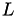and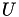are used directly to solve all equations at once. Due to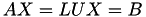with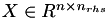being the solution matrix, first with the lower triangular matrixis solved and afterwards with the upper triangular matrix:

{
TDenseMatrix SOL( A->cols(), nrhs );
solve_option_t opt_LL( block_wise, unit_diag );
solve_option_t opt_UR( block_wise, general_diag );
RHS.copy_to( & SOL );
solve_lower_left( apply_normal, B.get(), & SOL, nullptr, fac_acc, opt_LL );
solve_upper_left( apply_normal, B.get(), & SOL, nullptr, fac_acc, opt_UR );
auto X = RHS.copy();
multiply( real_t(-1), apply_normal, A.get(), apply_normal, & SOL, real_t(1), X.get(), acc_exact );
cout << " |AX-B| = " << norm_F( X.get() ) << endl;
}

Sinceis computed with unit diagonal, this also has to be defined for solving. Furthermore, instead of A_inv, which also represents the inverse operator, the matrix A_copy has to be used since it holds the actual LU factors.

Remarks
Ifis computed as a symmetric matrix, LDL factorisation would have been applied. In this case the matrix solve method looks as:
solve_lower_left( apply_normal, A_copy.get(), & SOL, nullptr, fac_acc, opt_LL );
solve_diag_left( & SOL, apply_normal, A_copy.get(), nullptr, fac_acc, opt_UR );
solve_lower_left( apply_transposed, A_copy.get(), & SOL, nullptr, fac_acc, opt_LL );
In the hermitian case, apply_adjoint is to be used for the second solve_lower_left call.

The matrix approach yields to same residual as in the previous case. However, it may be more efficient in terms of runtime than the vector approach since it can exploit data locality much better.

However, the direct vector approach scales better with the number of cores. Hence, depending on your CPU configuration, this approach may be faster. Of course, the number of RHSs should be reasonably large for a good parallel scaling.

# The Plain Program

#include <iostream>
#include <string>
#include <boost/format.hpp>
#include <tbb/parallel_for.h>
#include "hlib.hh"
using namespace std;
using boost::format;
using namespace HLIB;
using real_t = HLIB::real;
using fnspace_t = TConstFnSpace;
namespace
{
class TMyRHS : public TBEMFunction< real_t >
{
private:
const double _alpha, _beta;
public:
TMyRHS ( const double alpha,
const double beta )
: _alpha( alpha )
, _beta( beta )
{}
// actual RHS function
virtual real_t
eval ( const T3Point & pos,
const T3Point & ) const
{
return real_t( sin( 0.5 * pos.x() * cos( _alpha * 0.25 * pos.z() ) ) * cos( _beta * pos.y() ) );
}
};
}
int
main ( int argc, char ** argv )
{
string gridfile = "grid.tri";
double eps = 1e-4;
double fac_eps = 1e-6;
if ( argc > 1 )
gridfile = argv;
try
{
INIT();
TAutoBSPPartStrat part_strat;
TBSPCTBuilder ct_builder( & part_strat );
TBCBuilder bct_builder;
auto grid = read_grid( gridfile.c_str() );
auto fnspace = make_unique< fnspace_t >( grid.get() );
auto coord = fnspace->build_coord();
auto ct = ct_builder.build( coord.get() );
auto bct = bct_builder.build( ct.get(), ct.get(), & adm_cond );
slpbf_t bf( fnspace.get(), fnspace.get() );
TBFCoeffFn< slpbf_t > coeff_fn( & bf,
bct->row_ct()->perm_i2e(),
bct->col_ct()->perm_i2e() );
TACAPlus< real_t > lrapx( & coeff_fn );
TTruncAcc acc = fixed_prec( eps );
TDenseMBuilder< real_t > h_builder( & coeff_fn, & lrapx );
auto A = h_builder.build( bct.get(), unsymmetric, acc, & progress );
const size_t nrhs = 500;
TQuadBEMRHS< fnspace_t, real_t > rhs_build( 4 );
TDenseMatrix RHS( fnspace->n_indices(), nrhs );
tbb::parallel_for( size_t(0), nrhs,
[&RHS,&rhs_build,&fnspace] ( const size_t i )
{
TMyRHS rhsfn( 100 * drand48() - 50,
100 * drand48() - 50 );
auto b = rhs_build.build( fnspace.get(), & rhsfn );
auto rhs_i = RHS.blas_rmat().column( i );
BLAS::copy( cptrcast( b.get(), TScalarVector )->blas_rvec(), rhs_i );
} );
RHS.permute( ct->perm_e2i(), nullptr );
auto A_copy = A->copy();
TTruncAcc fac_acc( fac_eps, 0.0 );
auto A_inv = factorise_inv( A_copy.get(), fac_acc, & progress );
{
TDenseMatrix SOL( A->cols(), nrhs );
tbb::parallel_for( size_t(0), nrhs,
[&RHS,&SOL,&A,&A_inv,fac_eps] ( const size_t i )
{
BLAS::Vector< real_t > rhs_i( RHS.blas_rmat().column( i ) );
BLAS::Vector< real_t > sol_i( SOL.blas_rmat().column( i ) );
TScalarVector b( A->row_is(), rhs_i );
TScalarVector x( A->col_is(), sol_i );
solve( A.get(), & x, & b, A_inv.get() );
} );
auto X = RHS.copy();
multiply( real_t(-1), apply_normal, A.get(), apply_normal, & SOL, real_t(1), X.get(), acc_exact );
cout << " |AX-B| = " << norm_F( X.get() ) << endl;
}
{
TDenseMatrix SOL( A->cols(), nrhs );
tbb::parallel_for( size_t(0), nrhs,
[&RHS,&SOL,&A,&A_inv] ( const size_t i )
{
BLAS::Vector< real_t > rhs_i( RHS.blas_rmat().column( i ) );
BLAS::Vector< real_t > sol_i( SOL.blas_rmat().column( i ) );
TScalarVector b( A->row_is(), rhs_i );
TScalarVector x( A->col_is(), sol_i );
A_inv->apply( & b, & x );
} );
auto X = RHS.copy();
multiply( real_t(-1), apply_normal, A.get(), apply_normal, & SOL, real_t(1), X.get(), acc_exact );
cout << " |AX-B| = " << norm_F( X.get() ) << endl;
}
{
TDenseMatrix SOL( A->cols(), nrhs );
solve_option_t opt_LL( block_wise, unit_diag );
solve_option_t opt_UR( block_wise, general_diag );
RHS.copy_to( & SOL );
solve_lower_left( apply_normal, A_copy.get(), & SOL, nullptr, fac_acc, opt_LL );
solve_upper_left( apply_normal, A_copy.get(), & SOL, nullptr, fac_acc, opt_UR );
auto X = RHS.copy();
multiply( real_t(-1), apply_normal, A.get(), apply_normal, & SOL, real_t(1), X.get(), acc_exact );
cout << " |AX-B| = " << norm_F( X.get() ) << endl;
}
DONE();
}
catch ( Error & e )
{
cout << "HLIB::error : " << e.what() << endl;
}
catch ( std::exception & e )
{
cout << "std::exception : " << e.what() << endl;
}
return 0;
}